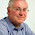# SAS : Brier Score for Model Calibration

The Brier score is an important measure of calibration i.e. the mean squared difference between the predicted probability and the actual outcome.
Lower the Brier score is for a set of predictions, the better the predictions are calibrated.
1. If the predicted probability is 1 and it happens, then the Brier Score is 0, the best score achievable.
2. If the predicted probability is 1 and it does not happen, then the Brier Score is 1, the worst score achievable.
3. If the predicted probability is 0.8 and it happens, then the Brier Score is (0.8-1)^2 =0.04.
4. If the predicted probability is 0.2 and it happens, then the Brier Score is (0.2-1)^2 =0.64.
5. If the predicted probability is 0.5, then the Brier Score is (0.5-1)^2 =0.25, irregardless of whether it happens.
By specifying fitstat option in proc logistic, SAS returns Brier score and other fit statistics such as AUC, AIC, BIC etc.
proc logistic data=train;
model y(event="1") = entry/ outroc = rocstats;
score data=valid out=valpred fitstat;
run;Share
Related PostsDeepanshu founded ListenData with a simple objective - Make analytics easy to understand and follow. He has over 10 years of experience in data science. During his tenure, he has worked with global clients in various domains like Banking, Insurance, Private Equity, Telecom and Human Resource.

4 Responses to "SAS : Brier Score for Model Calibration"
1.Hi Deepanshu,

I tried getting Brier score using fitstat option but seems I didnt get it.. Can you please place a snapshot here? Do i need to add any other option to get this score.

1.Which version of SAS you are using? The fitstat option in score statement is available in SAS versions starting from SAS 9.3

2.hello,
how is it possible to perform easily a calibration plot (observed probabilities as a function of the expected one) for a logistic model ?
best regards

3.Great tips and very easy to understand. This will definitely be very useful for me when I get a chance to start my blog
calibrationtraining.strikingly.com

Next → ← Prev
Love this post? Support Us!